Forecast to Power Tutorial¶

This tutorial will walk through the process of going from Unidata forecast model data to AC power using the SAPM.

This tutorial requires pvlib >= 0.6.0.

Authors:

• Derek Groenendyk (@moonraker), University of Arizona, November 2015
• Will Holmgren (@wholmgren), University of Arizona, November 2015, January 2016, April 2016, July 2016, August 2018

Setup¶

These are just your standard interactive scientific python imports that you'll get very used to using.

In :
# built-in python modules
import datetime
import inspect
import os

import numpy as np
import pandas as pd

# plotting stuff
# first line makes the plots appear in the notebook
%matplotlib inline
import matplotlib.pyplot as plt
import matplotlib as mpl

# finally, we import the pvlib library
from pvlib import solarposition, irradiance, atmosphere, pvsystem, inverter, temperature
from pvlib.forecast import GFS, NAM, NDFD, RAP, HRRR
c:\users\kanderso\software\anaconda3\envs\pvlib-dev\lib\site-packages\pvlib\forecast.py:19: UserWarning: The forecast module algorithms and features are highly experimental. The API may change, the functionality may be consolidated into an io module, or the module may be separated into its own package.
'The forecast module algorithms and features are highly experimental. '

pvlib forecast module only includes several models. To see the full list of forecast models visit the Unidata website:

http://www.unidata.ucar.edu/data/#tds

In :
# Choose a location.
# Tucson, AZ
latitude = 32.2
longitude = -110.9
tz = 'US/Mountain'

Define some PV system parameters.

In :
surface_tilt = 30
surface_azimuth = 180 # pvlib uses 0=North, 90=East, 180=South, 270=West convention
albedo = 0.2
In :
start = pd.Timestamp(datetime.date.today(), tz=tz) # today's date
end = start + pd.Timedelta(days=7) # 7 days from today
In :
# Define forecast model
fm = GFS()
#fm = NAM()
#fm = NDFD()
#fm = RAP()
#fm = HRRR()
In :
# Retrieve data
forecast_data = fm.get_processed_data(latitude, longitude, start, end)

In :
Out:
temp_air wind_speed ghi dni dhi total_clouds low_clouds mid_clouds high_clouds
2020-07-07 06:00:00-06:00 29.454681 5.270410 0.000000 0.000000 0.000000 0.0 0.0 0.0 0.0
2020-07-07 09:00:00-06:00 27.087494 1.988913 287.162801 140.869429 215.705993 57.0 0.0 57.0 0.0
2020-07-07 12:00:00-06:00 24.950012 3.782271 573.731739 123.802033 458.864565 59.0 0.0 59.0 0.0
2020-07-07 15:00:00-06:00 37.233734 3.298288 926.616347 767.306217 217.396559 0.0 0.0 0.0 0.0
2020-07-07 18:00:00-06:00 53.850006 3.230239 446.504106 724.204396 85.436744 0.0 0.0 0.0 0.0

This is a pandas DataFrame object. It has a lot of great properties that are beyond the scope of our tutorials.

In :
forecast_data['temp_air'].plot();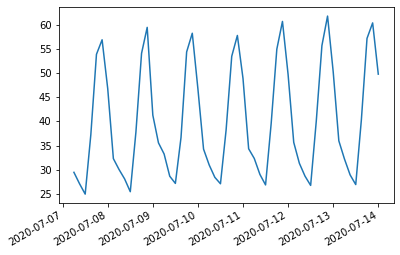Plot the GHI data. Most pvlib forecast models derive this data from the weather models' cloud clover data.

In :
ghi = forecast_data['ghi']
ghi.plot()
plt.ylabel('Irradiance ($W/m^{-2}$)');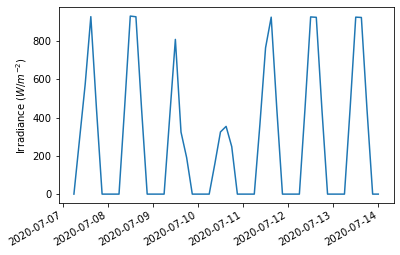Calculate modeling intermediates¶

Before we can calculate power for all the forecast times, we will need to calculate:

• solar position
• airmass
• angle of incidence
• POA sky and ground diffuse radiation
• cell and module temperatures

The approach here follows that of the pvlib tmy_to_power notebook. You will find more details regarding this approach and the values being calculated in that notebook.

Solar position¶

Calculate the solar position for all times in the forecast data.

The default solar position algorithm is based on Reda and Andreas (2004). Our implementation is pretty fast, but you can make it even faster if you install numba and use add method='nrel_numba' to the function call below.

In :
# retrieve time and location parameters
time = forecast_data.index
a_point = fm.location
In :
solpos = a_point.get_solarposition(time)
#solpos.plot()

The funny looking jump in the azimuth is just due to the coarse time sampling in the TMY file.

DNI ET¶

Calculate extra terrestrial radiation. This is needed for many plane of array diffuse irradiance models.

In :

#dni_extra.plot()
#plt.ylabel('Extra terrestrial radiation ($W/m^{-2}$)')

Airmass¶

Calculate airmass. Lots of model options here, see the atmosphere module tutorial for more details.

In :
airmass = atmosphere.get_relative_airmass(solpos['apparent_zenith'])

#airmass.plot()
#plt.ylabel('Airmass')

The funny appearance is due to aliasing and setting invalid numbers equal to NaN. Replot just a day or two and you'll see that the numbers are right.

POA sky diffuse¶

Use the Hay Davies model to calculate the plane of array diffuse sky radiation. See the irradiance module tutorial for comparisons of different models.

In :
forecast_data['dhi'], forecast_data['dni'], dni_extra,
solpos['apparent_zenith'], solpos['azimuth'])
#poa_sky_diffuse.plot()
#plt.ylabel('Irradiance ($W/m^{-2}$)')

POA ground diffuse¶

Calculate ground diffuse. We specified the albedo above. You could have also provided a string to the surface_type keyword argument.

In :

#poa_ground_diffuse.plot()
#plt.ylabel('Irradiance ($W/m^{-2}$)')

AOI¶

Calculate AOI

In :
aoi = irradiance.aoi(surface_tilt, surface_azimuth, solpos['apparent_zenith'], solpos['azimuth'])

#aoi.plot()
#plt.ylabel('Angle of incidence (deg)')

Note that AOI has values greater than 90 deg. This is ok.

POA total¶

In :

plt.ylabel('Irradiance ($W/m^{-2}$)')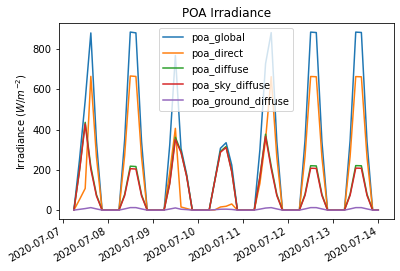Cell temperature¶

Calculate pv cell temperature

In :
ambient_temperature = forecast_data['temp_air']
wnd_spd = forecast_data['wind_speed']
thermal_params = temperature.TEMPERATURE_MODEL_PARAMETERS['sapm']['open_rack_glass_polymer']
pvtemp = temperature.sapm_cell(poa_irrad['poa_global'], ambient_temperature, wnd_spd, **thermal_params)

pvtemp.plot()
plt.ylabel('Temperature (C)');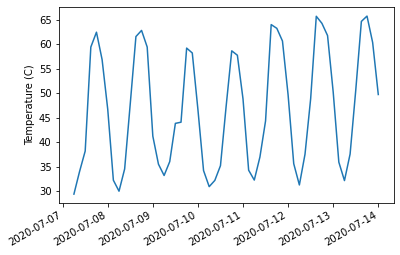DC power using SAPM¶

Get module data from the web.

In :
sandia_modules = pvsystem.retrieve_sam('SandiaMod')

Choose a particular module

In :
sandia_module
Out:
Vintage                                                          2009
Area                                                            1.701
Material                                                         c-Si
Cells_in_Series                                                    96
Parallel_Strings                                                    1
Isco                                                          5.09115
Voco                                                          59.2608
Impo                                                          4.54629
Vmpo                                                          48.3156
Aisc                                                         0.000397
Aimp                                                         0.000181
C0                                                            1.01284
C1                                                         -0.0128398
Bvoco                                                        -0.21696
Mbvoc                                                               0
Bvmpo                                                       -0.235488
Mbvmp                                                               0
N                                                              1.4032
C2                                                           0.279317
C3                                                           -7.24463
A0                                                           0.928385
A1                                                           0.068093
A2                                                         -0.0157738
A3                                                          0.0016606
A4                                                          -6.93e-05
B0                                                                  1
B1                                                          -0.002438
B2                                                          0.0003103
B3                                                         -1.246e-05
B4                                                           2.11e-07
B5                                                          -1.36e-09
DTC                                                                 3
FD                                                                  1
A                                                            -3.40641
B                                                          -0.0842075
C4                                                           0.996446
C5                                                           0.003554
IXO                                                           4.97599
IXXO                                                          3.18803
C6                                                            1.15535
C7                                                          -0.155353
Notes               Source: Sandia National Laboratories Updated 9...

Run the SAPM using the parameters we calculated above.

In :
airmass, aoi, sandia_module)

sapm_out[['p_mp']].plot()
plt.ylabel('DC Power (W)');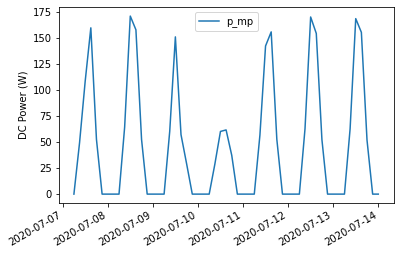AC power using SAPM¶

Get the inverter database from the web

In :
sapm_inverters = pvsystem.retrieve_sam('sandiainverter')

Choose a particular inverter

In :
sapm_inverter = sapm_inverters['ABB__MICRO_0_25_I_OUTD_US_208__208V_']
sapm_inverter
Out:
Vac                          208
Pso                      2.08961
Paco                         250
Pdco                     259.589
Vdco                          40
C0                      -4.1e-05
C1                      -9.1e-05
C2                      0.000494
C3                     -0.013171
Pnt                        0.075
Vdcmax                        50
Idcmax                   6.48972
Mppt_low                      30
Mppt_high                     50
CEC_Date                     NaN
CEC_Type     Utility Interactive
Name: ABB__MICRO_0_25_I_OUTD_US_208__208V_, dtype: object
In :
p_ac = inverter.sandia(sapm_out.v_mp, sapm_out.p_mp, sapm_inverter)

p_ac.plot()
plt.ylabel('AC Power (W)')
plt.ylim(0, None);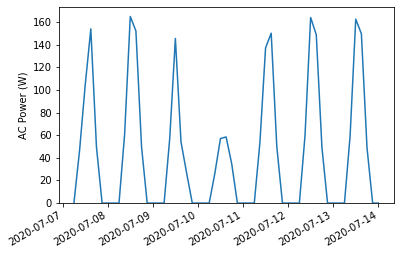Plot just a few days.

In :
p_ac[start:start+pd.Timedelta(days=2)].plot();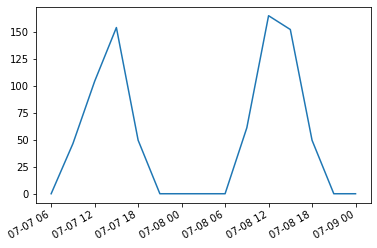Some statistics on the AC power

In :
p_ac.describe()
Out:
count     55.000000
mean      44.950796
std       57.381541
min       -0.075000
25%       -0.075000
50%       26.079967
75%       58.926306
max      164.985484
dtype: float64
In :
p_ac.index.freq
In :
# integrate power to find energy yield over the forecast period
p_ac.sum() * 3
Out:
7416.881315701111
In [ ]: